# Regression Learner App

February 18, 2022

`lang: en_US`

Regression is a statistical technique used in finance, investing, and other fields to assess the degree and nature of a relationship between a dependent variable and a set of independent factors. In MATLAB, the regression learner app will provide an interactive way to make a regression model. Regression Learner is used for training predictive models such as linear regression analysis, regression trees, Gaussian linear regression, support vector machine (SVM), and tree-based ensemble. Analyzing our dataset, selecting features, establishing evaluation strategies, and information findings add to training sets. It is also possible to save a trained model to the workspace and then use it with new data.

To do regression analysis in the regression learner app, follow the steps below.

• In the apps tab in the Machine Learning group, click on Regression Learner.
• Import data
• Choose independent variables, dependent variables, and validation scheme.
• Choose model type.
• Evaluate the performance of the model.
• Exporting of a model.

The information regarding the car's mileage is included in the data utilized for the analysis. There are five distinct characteristics of data. Out of the five features, the mileage is the output feature, while the other four are input features. As a result, we may deduce that all features are numerical, and we must design a regression model for this data set. HP, volume, speed, and weight are independent factors in this data set, while mileage is a dependent variable. We'll create a model to forecast car mileage. The Cars file can be downloaded from here.

## Regression Learner App

Fundamentally, the Regression Learner application enables you to interactively construct a prediction model without actually writing code and then measure their precision. In a couple of seconds, you may examine the execution of various regression models and parameters. To get started, go to the app section in MATLAB and select regression learner.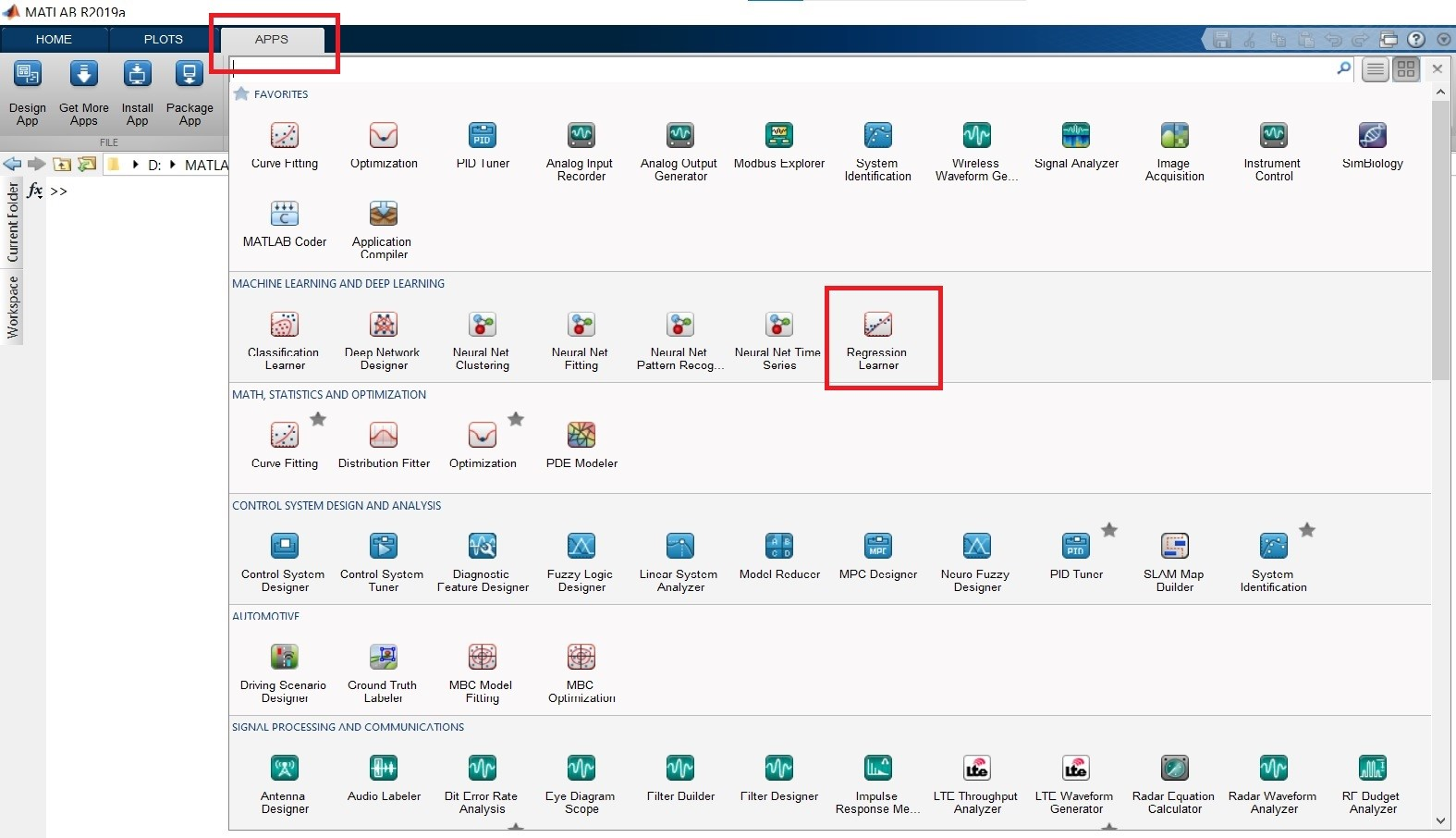Way to Regression Learner App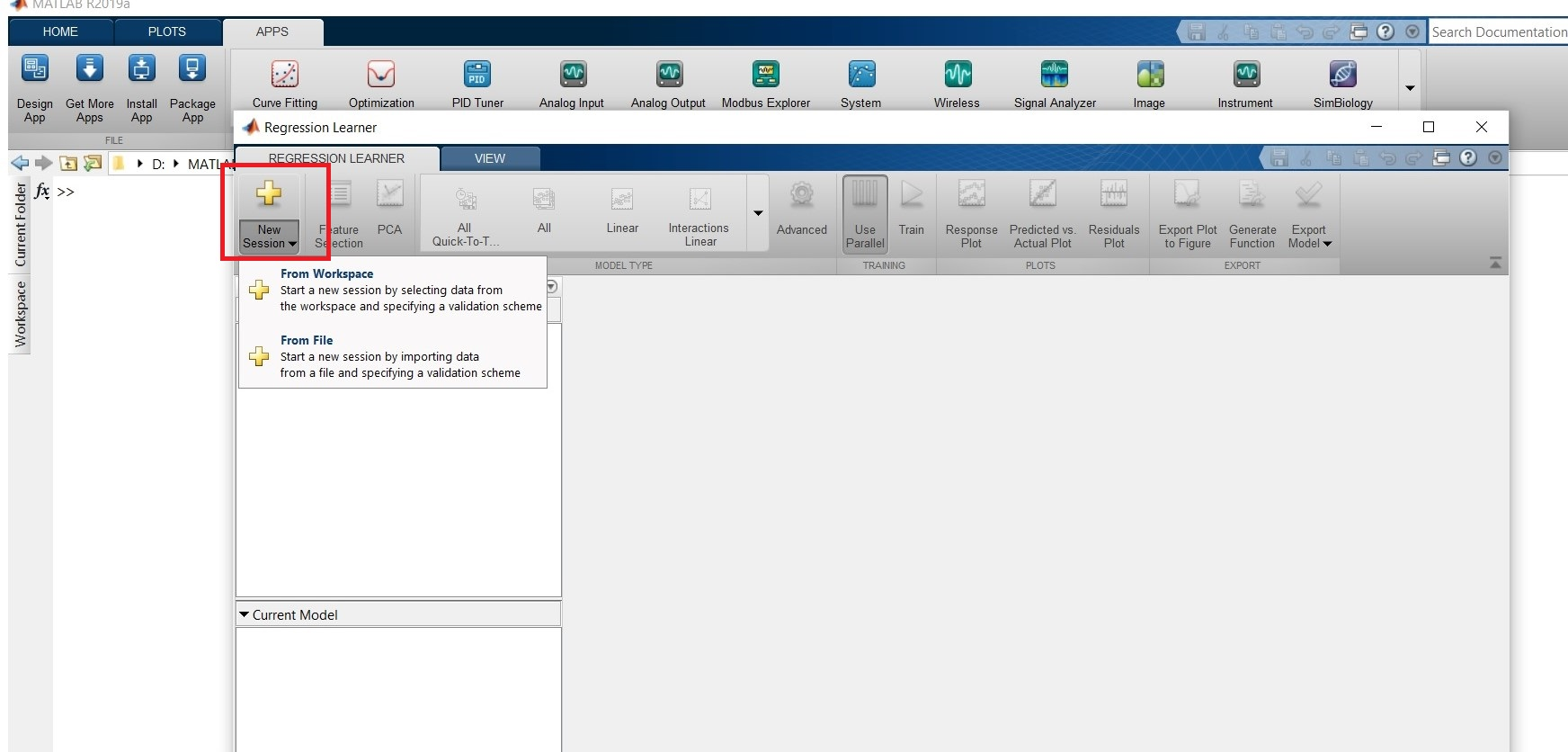Importing of Data

Start a session by selecting data from the workspace or file under the new session.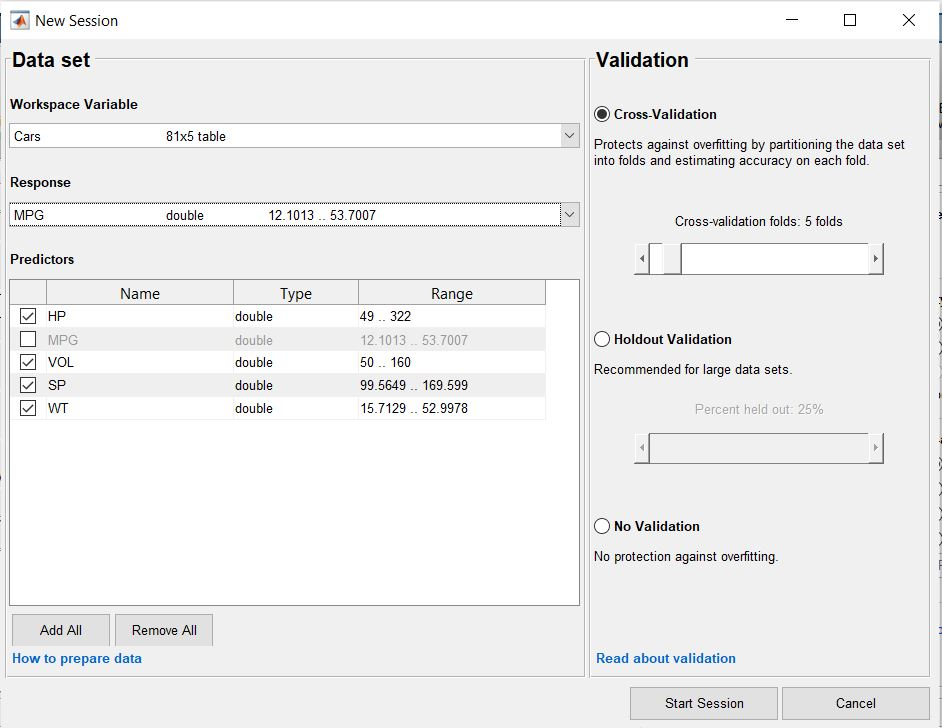Session

From the data set option choose independent and dependent features. Examine the roles that the app assigns to variables based on the data type. All other variables are predictors, and the software tries to find a suitable response variable. If necessary, make changes to the options. To test the predicted accuracy of the estimated model, choose one validation method. Validation lets you identify the optimal model by estimating predictive accuracy on new data. Overfitting is avoided with validation. Validate accuracy is worsened by a model that is excessively flexible and overfitted. Before developing any results, choose a method to examine all of the concepts in the session. The cross-validation methodology provides a good assessment of the ultimate model's forecast validity when trained on the entire data set. Repeated fits are required, but the solution makes optimum use of all data; therefore, it is suitable for smaller data sets.

Validation of Holdouts Using the slider control, select a proportion of the data to utilize as a validation set. The software uses the training set to train a model and the validation set to evaluate its performance. Holdout validation is only applicable for big data sets because the validation system is based on only a subset of the data. The whole data set is used to train the final model. If there is no validation, it signifies there is no safeguard against overfitting. The app uses all of the data for training and computes the error rate using the same data. We obtain an inflated estimate of the model's performance on fresh data if we don't have any test data. The training sample accuracy will almost certainly be excessively high, but the predicted accuracy will almost certainly be lower. After selection, we can start the session. To examine all the regression model possibilities, simply click on the Model Type section of the Regression Learner tab to expand the list of regression models. The gallery's options present beginning points with various parameters that can be used to solve various regression problems.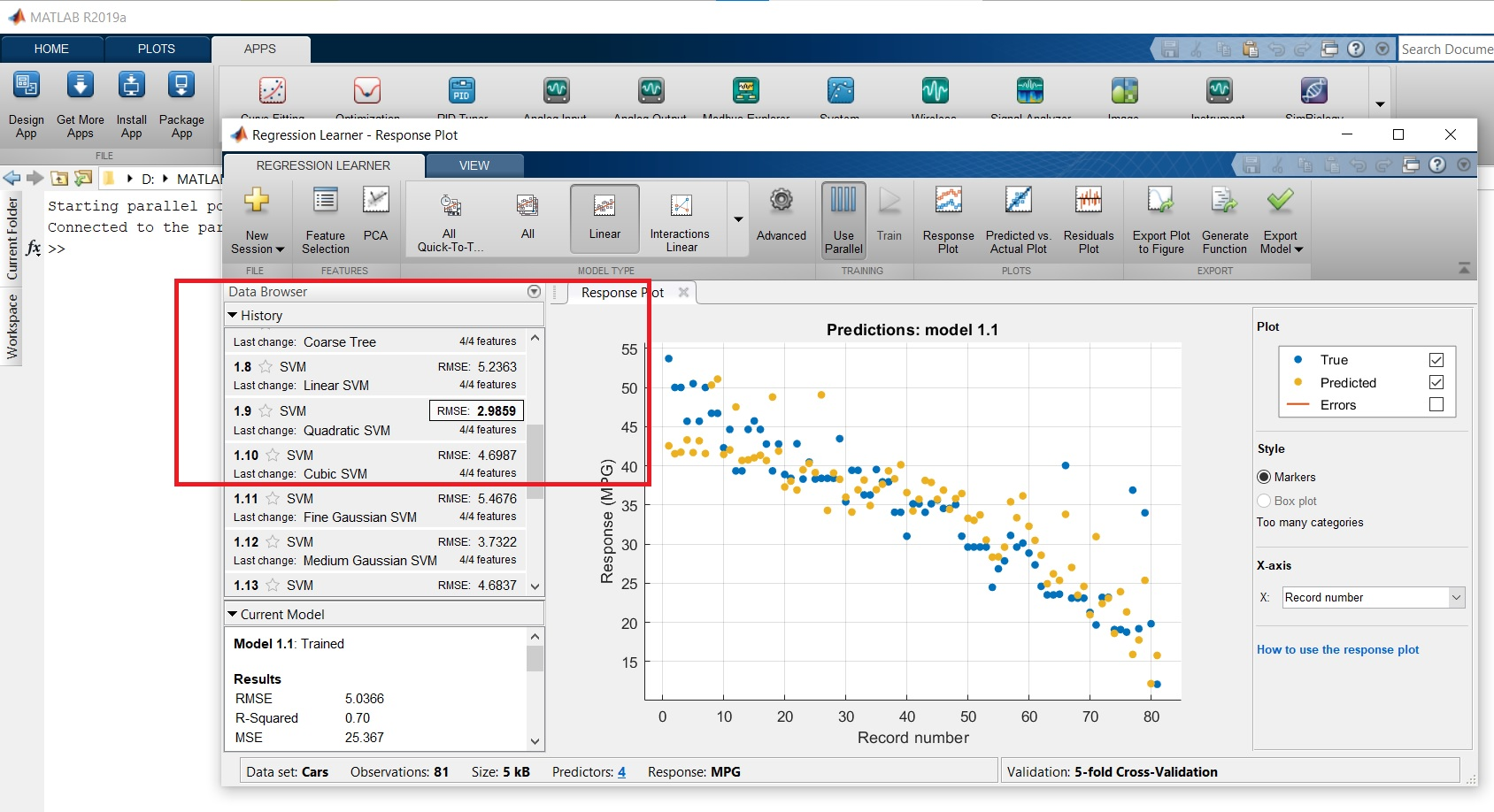Analysis after training

We can determine which model does have the best final record after training a model in Regression Learner by looking at the History list. A box is drawn around the highest score. This score represents the root mean square error (RMSE) on the validation data. The score predicts how well the trained model will perform with new data. Make use of the score to assist you in selecting the most appropriate model. In the Current Model pane, look up model statistics and use them to analyze and assess models. On the validation set, the statistics are computed.

## Model Performance in Regression Learner

Look at the regression model findings on the response plot. The response plot shows the anticipated response vs. record number after you've trained a regression model. These predictions are based on the held-out observations when using holdout or cross-validation. To put it another way, each prediction is based on a model developed without the use of the relevant observation.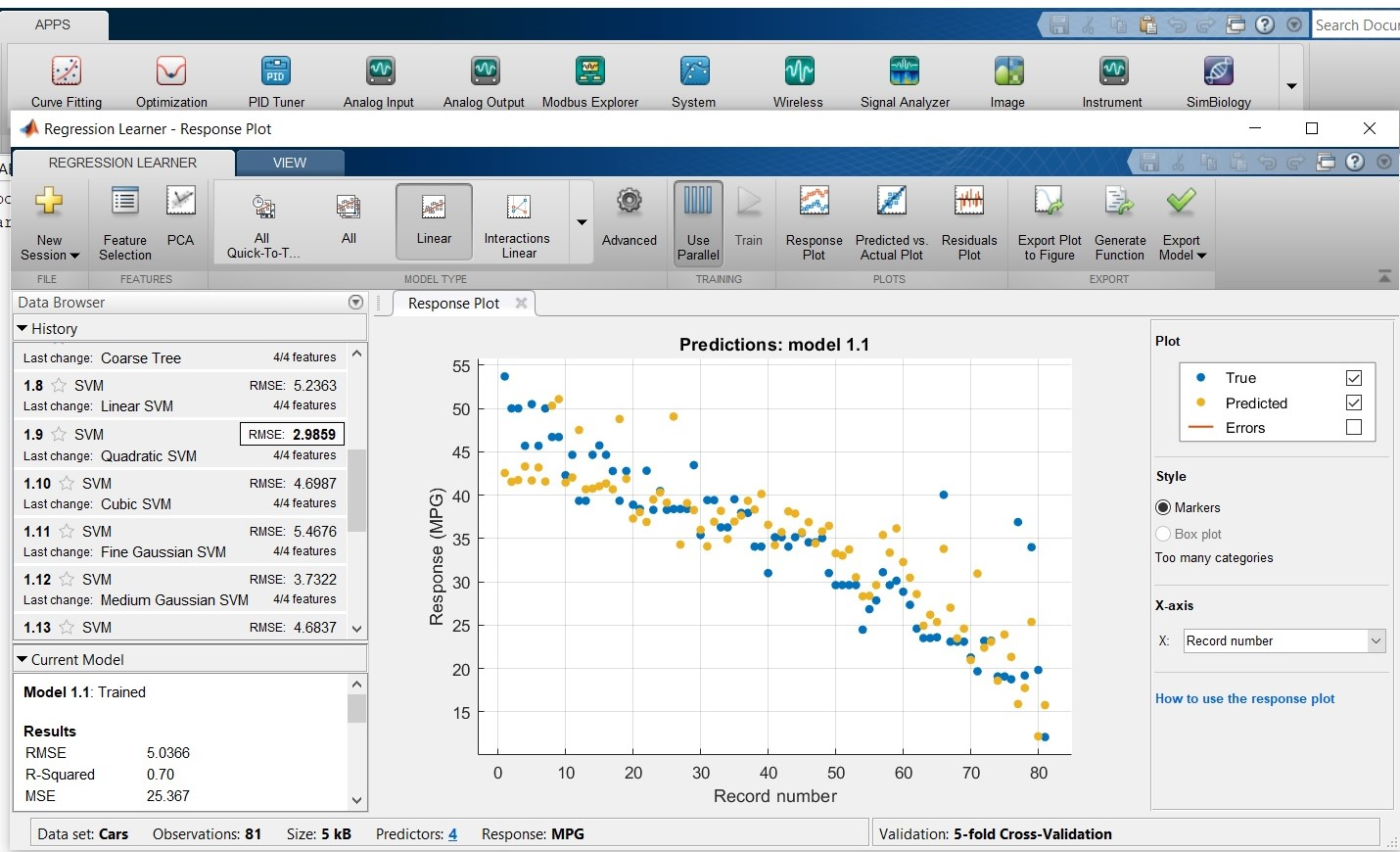Response Plot

To check model performance, look at the Predicted vs. Actual graphic below. Use this graph to see how well the regression model predicts different response values.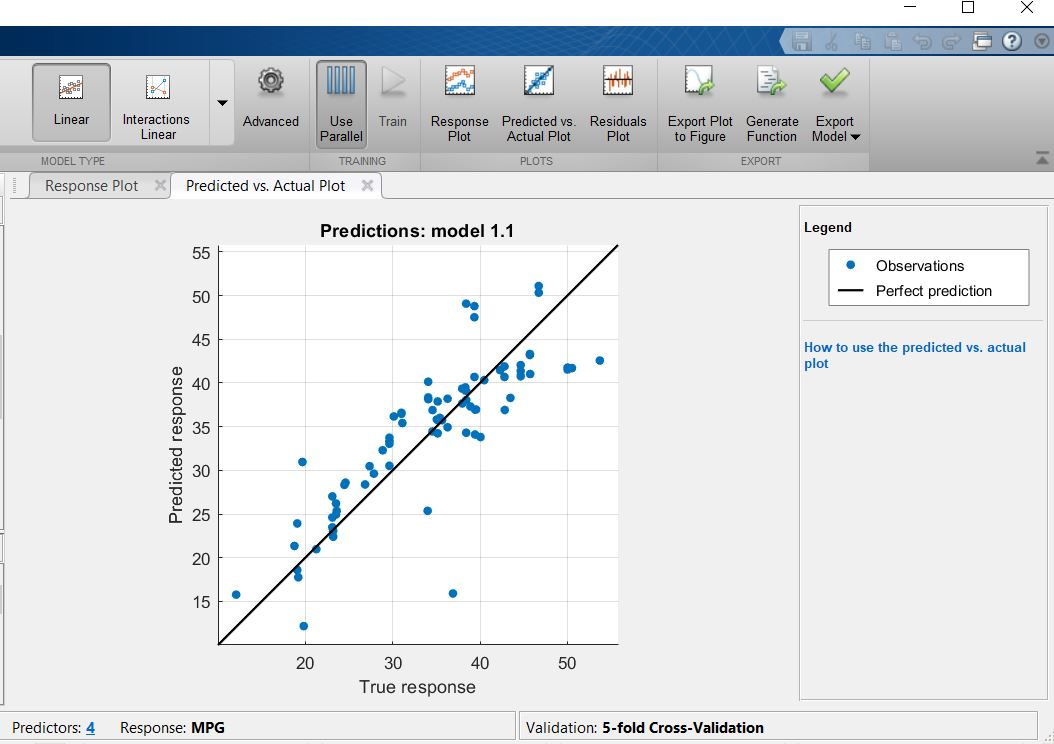predicted vs. actual plot

The residual plot will show the difference between actual and predicted entries for each training observation.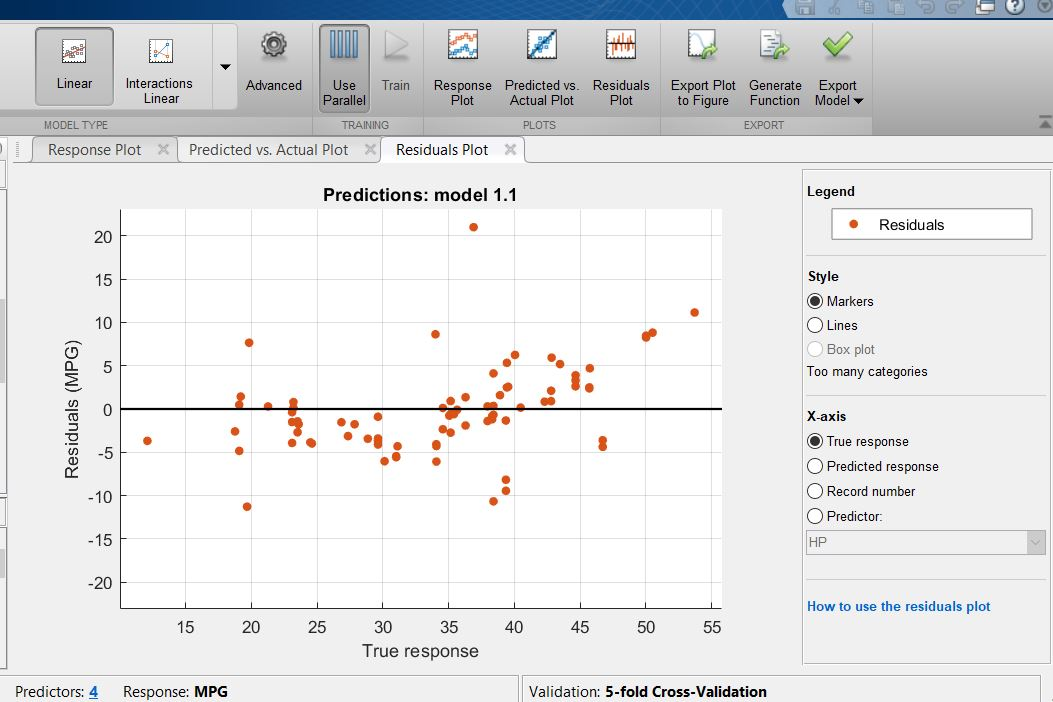Residuals Plot

We can export our best model to the workspace after creating interactive regression models in the Regression Learner app. Then we can apply the trained model to new data to make predictions.

## Conclusion

• We'll go through a step-by-step regression analysis with the Regression Learner App. Importing and exploring data, selecting features, specifying validation schemes, training models, and evaluating outcomes will all be straightforward and quick with this app.
• Linear regression models, regression trees, Gauss process regression models, vector support vehicles, and regression tree complexes can be automated trained to find the optimal regression model type. We can export the model to the workspace or produce the MATLAB code to reuse it with new data or do a programmatic regression.

Ready to take your MATLAB skills to the next level? Look no further! At MATLAB Helper, we've got you covered. From free community support to expert help and training, we've got all the resources you need to become a pro in no time. If you have any questions or queries, don't hesitate to reach out to us. Simply post a comment below or send us an email at [email protected].

And don't forget to connect with us on LinkedIn, Facebook, and Subscribe to our YouTube Channel! We're always sharing helpful tips and updates, so you can stay up-to-date on everything related to MATLAB. Plus, if you spot any bugs or errors on our website, just let us know and we'll make sure to fix it ASAP.

Ready to get started? Book your expert help with Research Assistance plan today and get personalized assistance tailored to your needs. Or, if you're looking for more comprehensive training, join one of our training modules and get hands-on experience with the latest techniques and technologies. The choice is yours – start learning and growing with MATLAB Helper today!

Education is our future. MATLAB is our feature. Happy MATLABing!

Insert Content Template or Symbol

Greetings from Anish !!!. I graduated from Veermata Jijabai Technological Institute in 2019 with a Master's degree in Control System. I'm obsessed with all tech-related things, and I'd like to succeed in a stimulating and challenging environment that will provide me with opportunities for advancement.

#### MATLAB Helper ®

Use Website Chat or WhatsApp at +91-8104622179